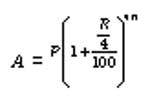## Numerical Ability/Quantitative Aptitude: Simple & Compound Interest

### Simple Interest:

It is the sum that is paid by the borrower to the lender for using the money for a specific time period. The money borrowed is called the Principal. The rate at which the interest is calculated on the principal is called the Rate of Interest. The time for which the money is borrowed is Time and the total sum of principal and interest is called the Amount.
Simple Interest

If P = Principal, R = Rate per cent per annum T = Number of years, SI = Simple Interest and A = Amount. Then,Here, the interest is calculated on the original principal ie, the principal to calculate the interest remains constant throughout the time period. The interest earned on the principal is not taken into account for the purpose of calculating interest for later years.

### Compound Interest:

In compound interest, the interest is added to the principal at the end of each period and the amount thus obtained becomes the principal for the next period. The process is repeated until the end of the specified time.

If P = Principal,

R = Rate percent per annum

Time = Number of years,

CI = Compound Interest

A = Amount. Then,

When the interest is compounded annually#### Important Formulae

1. If the rate of interest differs from year to year ie, R1 in the first year, R2 in the second year, R3 in the third year.

Then2. When the principal changes every year, we say that the interest is compounded annually. Then,3. When the principal changes as per every six months, we say that the interest is compounded half-yearly or semi-annually. Then,4. When the principal changes every three months, we say that the interest is compounded quarterly. Then,5. When the principal changes after every month, we say that the interest is compounded monthly. Then,6. When the interest is compounded annually but time is in fraction say a year.7. The difference between the simple interest and compound interest for 2 years (or terms) is given by the formulaWhere D is the difference, P is the principal and R is the rate of interest.

8. Present worth of x ` due n years, hence is given byClick here for more Simple Interest Problems: Simple Interest Tips and Shortcuts pdf

Click here for more Compound Interest Problems: Compound Interest Shortcut Tricks Pdf